Next: 7.5 Summary Up: 7. Numerical Considerations Previous: 7.3 Vectors in Discretized

# 7.4 Numerical Challenges Related to High-Voltage Devices

It was already stated earlier in this chapter that the derivatives on quantities in other mesh points are needed for assembly of the Jacobian matrix. The electric field in box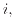for example, depends on the potentials in box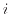and all neighboring boxes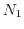-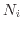: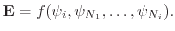(7.42)

Solving Poisson's equation, for example, only the electrostatic potential enters the equation via (7.6) and not the electric field. In this context, the electric field is only a post-processing quantity, the numerical solution process is not influenced by those dependencies. However, field dependent mobility models (see (4.14), for example) or generation terms like the impact-ionization generation (5.3) bring additional dependencies on quantities on neighboring vertices in the current equation and the generation term, respectively. This dependence on vector quantities, especially on the current density, results in couplings to many neighboring mesh points which generate many entries in the Jacobian matrix. The high number of non-zero entries in the system matrix often leads to poor performance or failure of iterative linear solvers . Additionally, strong non-linear relations, for example the exponential dependence used to model the impact-ionization rate, might lead to poor convergence of the Newton iteration . Many of the numerically problematic models are important in high-voltage and power devices and therefore have to be considered in the simulations.

An approach to overcome convergence issues is to calculate more intermediate solutions between the initial simulation step (equilibrium) and the desired operating point. This can be achieved by ramping the contact potentials step by step until the final value is achieved. Results from former steps are used as initial guesses for the Newton method in the next step. Decreasing the step size therefore improves the quality of the initial guess for the next step and finally for the desired operating point. Obviously a good balance between step size, robustness, and simulation time has to be found. Other approaches to overcome convergence issues are to tune the Newton procedure, for example, by changing parameters of the damping algorithm . This changes the calculation of the Newton update vectors and is often required to achieve convergence.

Numerical problems are frequently caused by the simulation mesh used. For mesh design a trade-off between accuracy, simulation time, and numerical stability has to be found. High convergence rates can be achieved by having a moderate number of well shaped elements . However, smaller elements usually lead to a higher resolution and therefore to a higher accuracy. On the other hand, the limited floating point precision in computer systems can result in numerical noise  which degrades the condition of the system matrix. For accurate results also a proper alignment of the elements regarding the direction of the current flow (see Fig. 7.7) is important. Near the channel area this often leads to poorly shaped thin elements having small internal angles. Creating a mesh for the simulation of high-voltage devices that has good numerical properties and delivers accurate results is very challenging.

Applications with special demands on the numerics of TCAD simulation tools are break-down, electro-static discharge (ESD), and snap-back simulations [275,221]. A case study on snap-back simulations in a simplified smart power device structure is demonstrated in Section 5.3. Difficulties arise in such simulations from the strongly field dependent behavior and the physically unstable operating points. Physical quantities undergo strong variations near breakdown and snap-back. Effects, for example, the base pushout phenomenon, completely change the operation state of the device. This also impacts the convergence of the iterative solver, since the state transition leads to strong changes in the conductivity, of the current path, and of the carrier concentrations. The different states of the devices before and after snap-back additionally result in multivalued I/V curves. The boundary conditions therefore have to be selected appropriately to avoid unintentional transitions between the branches of the I/V curve. Special curve-tracing algorithms have been suggested to deal with these problems [219,220].Next: 7.5 Summary Up: 7. Numerical Considerations Previous: 7.3 Vectors in Discretized

O. Triebl: Reliability Issues in High-Voltage Semiconductor Devices